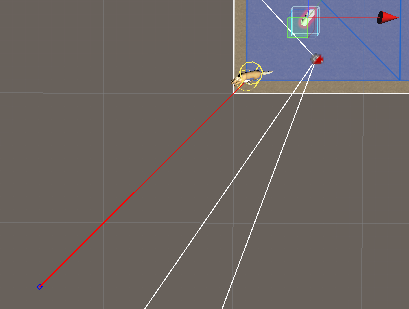# How to check the RichAI path is reachable?

Hi,

My NPC moves by RichAI.SearchPath().
By the way, I need to know whether the path is reachable or not before NPC starts to move.

How do I make it?

Thank you``````bool IsPathReachable(Vector3 targetPosition)
{
var node1 = AstarPath.active.GetNearest(m_Transform.position);
var node2 = AstarPath.active.GetNearest(targetPosition);

return PathUtilities.IsPathPossible(node1.node, node2.node);
}
``````

The horse is evading from the target.
targetPosition is the end point of a red line which the horse try to evade.

I want to check the point is reachable or not, so I wrote the above script.
But the script always returns true although the end point is on out of ground.

Ah. So the code really checks for “is the closest point on the navmesh to point A reachable from the closest point on the navmesh to point B”.
In this case the point is simply not on the navmesh, so you also need to account for that.
You can do something like:

``````var node1 = AstarPath.active.GetNearest(m_Transform.position);
if (Vector3.Distance(node1.position, m_Transform.position) > 0.5f) return false;
``````

And similarly for the other GetNearest call.
It’s useful to allow some margin for error (in this case 0.5 world units).

``````1. bool IsPathReachable(Vector3 targetPosition)
2. {
3.     var node1 = AstarPath.active.GetNearest(m_Transform.position).node;
4.     if (Vector3.Distance(m_Transform.position, (Vector3)node1.position) > 0.5f)
5.         return false;

6.     var node2 = AstarPath.active.GetNearest(targetPosition).node;
7.     if (Vector3.Distance(targetPosition, (Vector3)node2.position) > 0.5f)
8.         return false;

9.     return PathUtilities.IsPathPossible(node1, node2);
10. }
``````

I modified the script as above.
Now, it always returns false in line 5!

m_Transform.position is the horse’s position. Now, The horse cannot move anywhere with your suggestion even it is located in the middle of level.

You should check the ‘position’ property of the value that GetNearest returns, not the position of the node.

``````var nn1 = AstarPath.active.GetNearest(m_Transform.position);
var node1 = nn1.node;
if (Vector3.Distance(m_Transform.position, nn1.position) > 0.5f)
return false;
``````

Note that this will also cause it to return false if you check for a point say 0.5 meters above the navmesh. So you may want to check the distance only along the X and Z axes.

``````bool IsPathReachable(Vector3 targetPosition)
{
var nn1 = AstarPath.active.GetNearest(m_Transform.position);
if (Vector3.Distance(m_Transform.position, nn1.position) > 0.5f)
return false;

var nn2 = AstarPath.active.GetNearest(targetPosition);
if (Vector3.Distance(targetPosition, nn2.position) > 0.5f)
return false;

return PathUtilities.IsPathPossible(nn1.node, nn2.node);
}
``````

Ok, the script works now! Thank you.